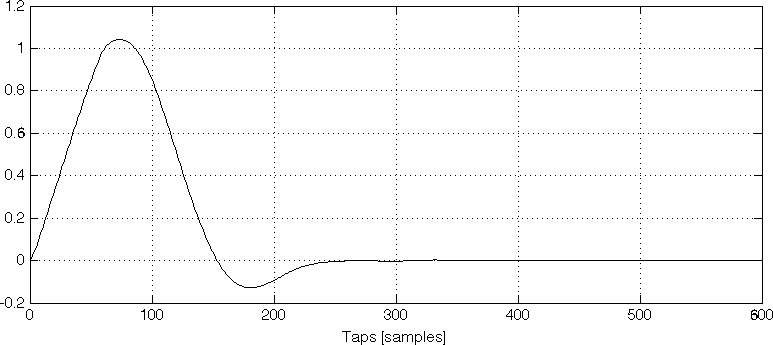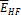5.1.2 Complex low-delay filter bank analysis

26.4453GPPCodec for Enhanced Voice Services (EVS)Detailed algorithmic descriptionRelease 15TS

5.1.2.1 Sub-band analysis

The audio signal is decomposed into complex valued sub-bands by a complex modulated low delay filter bank (CLDFB). Depending on the input sampling rate , the CLDFB generates a time-frequency matrix of 16 time slots and sub-bands where the width of each sub-band is 400 Hz.

The analysis prototype is an asymmetric low-pass filter with an adaptive length depending on. The length of is given by meaning that the filter spans over 10 consecutive blocks for the transformation. The prototype of the LP filter has been generated for 48 kHz. For other input sampling rates, the prototype is obtained by means of interpolation so that an equivalent frequency response is achieved. Energy differences in the sub-band domain caused by different transformation lengths are compensated for by an appropriate normalization factors in the filter bank. The following figure shows the plot of the LP filter prototype for of 48 kHz.Figure 4 : Impulse response of CLDFB prototype filter with 600 taps for 48 kHz sample rate

The filter bank operation is described in a general form by the following formula:

(2)

where and are the real and the imaginary sub-band values, respectively, is the sub-band time index with , index is defined as , is the modulation offset of andis the sub-band index with .

As the equations show, the filter bank is comparable to a complex MDCT but with a longer overlap towards the past samples. This allows for an optimized implementation of CLDFB by adopting DCT-IV and DST-IV frameworks.

5.1.2.2 Sub-band energy estimation

The energy in the CLDFB domain is determined for each time index and frequency sub-band by

(3)

Furthermore, energy per-band is calculated by summing up the energy values in all time slots. That is

(1)

In case , additional high frequency energy valueis calculated for the frequency range from 8kHz to 16kHz by summing up over one frame which is delayed by one time slot.

(2)

is further scaled to an appropriate energy domain. In case the high bands are not active, is initialized to the maximum value.